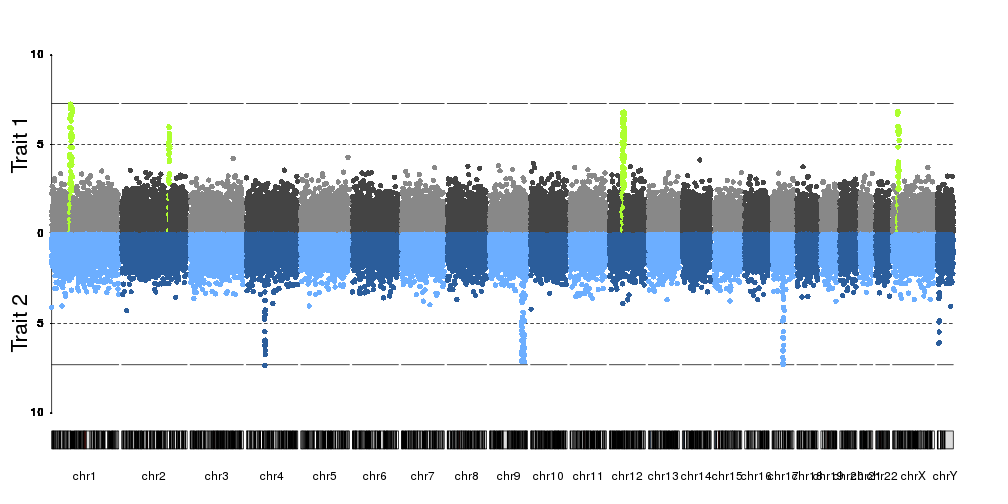Mirror Manhattan Plot
3
1
Entering edit mode
5.4 years ago
jennysjaarda ▴ 10

Does anyone know how to adapt the qqman Manhattan plot (or other function) to create a mirror image Manhattan plot as seen here (i.e. reverse the axis on the bottom plot): https://www.researchgate.net/figure/284888365_fig2_Fig-2-Manhattan-plot-of-the-Burmese-head-deformity-GWAS-and-SNPs-genotypes-within

qqman manhattan GWAS plot R • 4.9k views
0
Entering edit mode

Did you try to invert the ylim option. Intead of c(0,10) for example, try c(10,0).

0
Entering edit mode

They are called Miami plot. (Because of the reflection of buildings on Miami biscayne bay)

Related SO post:

2
Entering edit mode
2.7 years ago
bernatgel ★ 3.3k

To plot mirrored manhattan plots you can use kpPlotManhattan from the karyoploteR Bioconductor package.

kp <- plotKaryotype(plot.type=4)
kpAddLabels(kp, labels = "Trait 1", srt=90, pos=3, r0=0.5, r1=1, cex=1.8, label.margin = 0.025)
kpAxis(kp, ymin=0, ymax=10, r0=0.5)
kp <- kpPlotManhattan(kp, data=ds$snps, highlight = ds$peaks, r0=0.5, r1=1, ymax=10)
kpAddLabels(kp, labels = "Trait 2", srt=90, pos=3, r0=0, r1=0.5, cex=1.8, label.margin = 0.025)
kpAxis(kp, ymin=0, ymax=10, r0=0.5, r1=0, tick.pos = c(5,10))
kp <- kpPlotManhattan(kp, data=createDataset()\$snps, r0=0.5, r1=0, ymax=10, points.col = "2blues")1
Entering edit mode
17 months ago
DM ▴ 30

Hi,

You could do something similar to this

library(qqman)
par(mfrow=c(2,1))
par(mar=c(0,5,3,3))
manhattan(gwasResults,ylim=c(0,10),cex=2.2,cex.lab=2.5,font.lab=2,font.axis=2,cex.axis=1.6,las=2,font=4)
par(mar=c(5,5,3,3))
manhattan(gwasResults,ylim=c(10,0),cex=2.2,cex.lab=2.5,font.lab=2,font.axis=2,cex.axis=1.6,las=2,font=4,xlab="",xaxt="n")
dev.off()1
Entering edit mode
12 months ago
Jimbou ▴ 880

You can try a ggplot-tidyverse solution based on ggfastman.

library(tidyverse)
library(ggfastman)
data("gwas_data")
gwas_data %>%
mutate( gr= "Study 1") %>%
mutate(pvalue=log10(pvalue)) %>%
# rbind a second study with pvalues with other sign.
bind_rows(., mutate(., gr= "Study 2",
pvalue = -pvalue)) %>%
# plot the points
fast_manhattan(., build = "hg18", speed = "fast",log10p = F, dodge_x = T,pointsize = 2.1, pixels = c(1000,500)) +
geom_hline(data= . %>% group_by(gr) %>% slice(1), aes(yintercept = ifelse(pvalue>0, -log10(5e-08),log10(5e-08))),color ="deeppink") +
coord_flip()+
scale_y_continuous(expression(-log(italic(p))),breaks= seq(-90,80,10), labels = abs(seq(-90,80,10)), expand = c(0.01, 0))+
facet_wrap(~gr, scales = "free_x")+
ggplot2::guides(y = guide_axis(n.dodge = 2), x = guide_axis())+
theme_bw()